Courses

# Previous Year Questions - Periodic Classification of Elements Class 10 Notes | EduRev

## Class 10 : Previous Year Questions - Periodic Classification of Elements Class 10 Notes | EduRev

The document Previous Year Questions - Periodic Classification of Elements Class 10 Notes | EduRev is a part of the Class 10 Course Science Class 10.
All you need of Class 10 at this link: Class 10

Q.1. An element ‘X’ with electronic configuration (2, 8, 2) combines separately with two radicals, (NO3)- and (SO4)2-.
(i) Is ‘X’ a metal or a non-metal? Write the nature of its oxide
(ii) Write the formula of the compounds of ‘X’ formed by the combination of these radicals. Are these compounds covalent or electrovalent?    [AI 2017C]
Ans.
(i) X is a metal as it can lose 2 electrons from its valence shell. It forms basic oxides.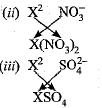‘X’ will form electrovalent compounds.

Q.2. Na, Mg and Al are the elements of the 3rd period of the Modern Periodic Table having group number 1, 2 and 13 respectively. Which one of these elements has the (a) highest valency, (b) largest atomic radius, and (c) maximum chemical reactivity? Justify your answer stating the reason for each.    [AI 2017]
Ans. (a) Al has highest valency equal to 3 because its electronic configuration is 2, 8, 3. It can lose 3 electrons to become stable.
(b) Na has largest atomic size because it has least effective nuclear charge as the atomic radius decreases when we move left to right in a period.
(c) Na is most reactive due to largest atomic size, it can lose electron easily due to attain the noble gas configuration.

Q.3. Write the names given to the vertical columns and horizontal rows in the Modern Periodic Table.
How does the metallic character of elements vary on moving down a vertical column? How does the size of atomic radius vary on moving left to right in a horizontal row? Given reason in support of your answer in the above two cases. [Delhi 2017]
Ans.
The vertical columns of periodic table are called groups.
The horizontal rows are called periods.
Metallic character: It increases down the group in a vertical column.
Reason: Effective nuclear charge decreases, as tendency to lose electrons increases on moving down the group because the attraction between the nucleus and the valence electron decreases.
Atomic radius: It decreases along left to right in a period.
Reason: It is because number of shells remain the same. Effective nuclear charge increases due to addition of one proton and one electron successively.

Q.4. An element ‘M’ has atomic number 12.
(i) Write its electronic configuration and valency.
(ii) Is ‘M’ a metal or non-metal? Give reason in support of your answer.
(iii) Write the formula and nature (acidic/basic) of the oxide of M.    [Delhi 2017C]
Ans. (i) Its electronic configuration 2, 8,2 and valency is 2.
(ii) ‘M’ is a metal as it can lose its 2 valence electrons to achieve the noble gas configuration.
(iii) The formula and nature of the oxide of M is MO and it forms basic oxide.

Q.5. An element P (atomic number 20) reacts with an element Q (atomic number 17) to form a compound. Answer the following questions giving reason: Write the position of P and Q in the Modern Periodic Table and the molecular formula of the compound formed when P reacts with Q.    [Delhi 2017]
Ans.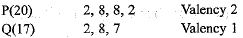Element P has 2 valence electrons and 4 shells, therefore, it belongs to group 2 and 4th period of periodic table Element Q has 7 valence electrons and 3 shells, therefore, it belongs to group 17 and 3rd period of periodic table.
The compound formed between P and Q will have formula PO2

Q.6. The atomic number of an element is 20.
(a) Write its electronic configuration and determine its valency.
(b) Is it a metal or a non-metal?
(c) Write the formula of its chloride.
(d) Is it more reactive or less reactive than Mg (atomic number 12)? Give reason for your answer. [Foreign 2017]
Ans. (a) Electronic configuration: 2, 8, 8, 2; valency: 2.
(b) It is a metal.
(c) XCl2 or CaCl2
(d) It is more reactive than Mg because it is larger in size, therefore has more tendency to lose electrons due to less effective nuclear charge.

Q.7. (a) Name the metals among the first five elements of the Modern Periodic Table.
(b) Write their chemical symbols.
(c) Write the formula of their oxides.    [CBSE 2016]
Ans. (a) Lithium and Beryllium
(b) Li and Be
(c) Li2O and BeO are formula of their oxides.

Q.8. Two elements X and Y have atomic number 11 and 16 respectively.
(i) Write the electronic configuration of both.
(ii) Which type of bond will they form?
(iii) Write the formula of the compound formed by their combination (in terms of X and Y).    [CBSE 2016]
Ans.
(i) Electronic configuration of X (2, 8, 1) and Y(2,8,6)
(ii) They will form ionic bond
(iii)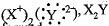X2Y is the formula of compound formed.

Q.9. An element ‘X’ belongs to 3rd period and group 16 of the Modern Periodic Table.
(а) Determine the number of valence electrons and the valency of ‘X’.
(b) Molecular formula of the compound when ‘X’ reacts with hydrogen and write its electron dot structure.
(c) Name the element ‘X’ and state whether it is metallic or non-metallic.    [Al 2016]
Ans. (a) ‘X’ has electronic configuration 2, 8, 6
∴ It has 6 valence electrons, therefore, it valency equal to 2.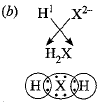(c) ‘X’ is Sulphur. It is non-metallic.

Q.10. An element ‘X’ (Atomic number 20) burns in presence of oxygen to form a basic oxide.
(a) Identify the element and write its electronic configuration.
(b) State its group number and period number in the Modern Periodic Table.
(c) Write a balanced chemical equation for the reaction when this oxide is dissolved in water.    [CBSE 2016]
Ans. (a) The element is calcium. Its electronic configuration 2, 8, 8, 2.
(b) It belongs to group 2 and 4th period.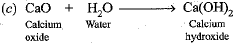Q.11. Out of the following elements
H (l); Be(4); N a ( l l ) ; Mg(12)
(a) Write the pair elements having similar chemical properties.
(b) State the group number of each pair.
(c) Name one other element belonging to each of these groups. [CBSE 2016]
Ans. (a) Be (4) and Mg (12)
H (1) and Li (3)
(b) 1st pair belongs to Group 2nd pair belongs to Group 1.
(c) Calcium belongs to Group 2, sodium belongs to Group 1.

Q.12. An element ‘X’ has mass number 35 and number of neutrons 18. Write atomic number and electronic configuration of ‘X’. Also write group number, period number and valency of ‘X’.     [AI 2016]
Ans. ‘X’ has mass number = 35
Number of neutrons =18
Number of protons = Atomic number = 35-18 = 17
Electronic configuration 2, 8, 7
It belongs to group 17 and 3rd period.
Its valency is equal to 1.

Q.13. An element ‘M’ with electronic configuration (2, 8, 2) combines separately with (NO4)3-, (SO4)2- and (PO4)3- radicals. Write the formula of the three compounds so formed. To which group and period of the Modern Periodic Table does the element ‘M’ belong? Will ‘M’ form covalent or ionic compounds? Give reason to justify your answer.    [Delhi 2016]
Ans.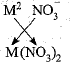‘M’ or Mg has 2 valence electrons, therefore, its valency is equal to 2.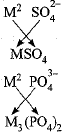It belongs to group 2nd and 3rd period of Modem Periodic Table.
‘M’ will form ionic compounds because it can lose 2 electrons easily to become stable.

Q.14. Which has larger atomic radius Ca (20) or K (19).    [CBSE 2016]
Ans. K (19) has larger atomic radius due to less effective number charge.

Q.15. Out of Li and K, which have stronger metallic character and why?    [CBSE 2016]
Ans. K because it has larger size and more tendency to lose electron.

Q.16. An element has atomic number 17 to which group and period closer it belong. [CBSE 2016]
Ans. Its electronic configuration is 2, 8, 7. It belongs to group 17 because it has 7 valence electrons. It belongs to 3rd period because it has 3 shalls.

Q.17. Calcium is an element with atomic number 20. Stating reason answer each of the following questions :
(i) Is calcium a metal or non-metal ?
(ii) Will its atomic radius be larger or smaller than that of potassium with atomic number 19 ?
(iii) Write the formula of its oxide.    [CBSE 2016]
Ans.
(i) Calcium is a metal.
The oxide of calcium viz., CaO is a basic oxide because it dissolves in water to give a base (alkali).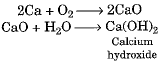(ii) Atomic radius of Ca is smaller than that of K. This is because, the next electron enters the same orbit as that of the last electron of K.
Electronic configuration of K : 2, 8, 8, 1
Electronic configuration of Ca : 2, 8, 8, 2
And because of the increased nuclear charge, the electrons in the last orbit are attracted with a greater force resulting in the decrease of atomic radius.
(iii) CaO is the formula of oxide of calcium.

Q.18. The elements 4Be, 12Mg and 20Ca, each having two valence electrons in their valence shells, are in periods 2, 3 and 4 respectively of the modern periodic table. Answer the following questions associated with these elements, giving reason in each case:
(a) In which group should they be?
(A) Which one of them is least reactive?
(c) Which one of them has the largest atomic size?    [AI 2015, 2014]
Ans. (a) They belong to group 2, since they have 2 valence electrons.
(b) ‘Be’ is least reactive because of more effective nucleus charge due to smaller size and least tendency to lose electrons.
(c) ‘Ca’ has the largest atomic size due to presence of 4 shells.

Q.19. Taking the example of an element of atomic num ber 16, exp lain how the electronic configuration of the atom of an element relates to its position in the Modern Periodic Table and how valency of an element is calculated on the basis of its atomic number.    [Al 2015]
Ans. S(16) has electronic configuration 2, 8, 6. It has 6 valence electrons. It belongs to Group 16. It has three shells, therefore, it belongs to 3rd period. It has 6 valence electrons. It can gain 2 electrons to complete its octet, therefore, its valency is equal to 2.

Q.20. Given below are some elements of the Modern Periodic Table. Atomic number of the element is given in the parentheses:
A(4), B(9), C(14), D(19), E(20)
(a) Select the element that has one electron in the outermost shell. Also write the electronic configuration of this element.
(b) Which two elements amongst these belong to the same group? Give reason for your answer.
(c) Which two elements amongst these belong to the same period? Which one of the two has bigger atomic radius?    [Al 2015]
Ans.
(a) D(19) = 2, 8, 8, 1
It has one valence electron.
(b) ‘A- and ‘E’ belong to same group because they have same number of valence electrons.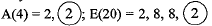(c) ‘A’ and ‘B’ belong to same period as they have same number of shells. A(4) = 2,2; B(9) = 2, 7. ‘A’ has bigger atomic size due to less effective nuclear charge.

Q.21. Na, Mg and Al are the elements of the same period of Modern Periodic Table having one, two and three valence electrons respectively. Which of these elements (i) has the largest atomic radius, (ii) is least reactive? Justify your answer stating reason for each case.    [Delhi 2015]
Ans. (i) Na has largest atomic radius because it has 11 protons and 11 electrons and least effective nuclear charge among these elements.
(ii) Al is least reactive because it is smallest in size, therefore, has most effective nuclear charge, least tendency to lose electrons.

Q.22. Two elements ‘P’ and ‘Q’ belong to the same period of the modern periodic table and are in Group-1 and Group-2 respectively. Compare their following characteristics in tabular form:
(a) The number of electrons in their atoms
(b) The sizes of their atoms
(c) Their metallic characters
(d) Their tendencies to lose electrons
(e) The formula of their oxides
(f) The formula of their chlorides    [Al 2015]
Ans.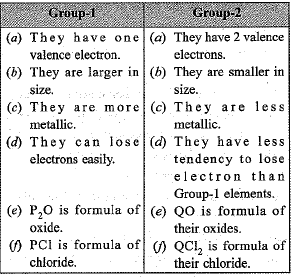Q.23. How many groups and periods are there in the modern periodic table ? How do the atomic size and metallic character of elements vary as we move :
(a) down a group and
(b) from left to right in a period.    [CBSE 2015]
Ans. There are 18 groups and 7 periods in the modem periodic table.
(a) On moving down a group, the atomic size increases and metallic character also increases.
(b) On moving from left to right in a period, the atomic size decreases and the metallic character also decreases.

Q.24. From the following elements :
4Be; 9F; 19K; 20Ca
(i) select the element having one electron in the outermost shell.
(ii) two elements of the same group.

Write the formula of and mention the nature of the compound formed by the union of 19K and element X(2, 8, 7).    [CBSE 2015]
Ans.
(i) 19K has one electron in the outermost shell. It has the electronic configuration :
2 , 8 , 8 , 1.
(ii) 4Be and 2oCa belong to group 2.
Both of them have two electrons in the outermost shell and therefore belong to group 2 (same group).
Formula of the compound formed by the union of 19K and X(2, 8, 7) is : KX.
Nature of the compound will be ionic because K(2, 8, 8,1) will donate one electron to X. This will complete the octet of both.

Q.1. (a) The modern periodic table has been evolved through the early attempts of Dobereiner, Newland and Mendeleev. List one advantage and one limitation of all the three attempts.
(b) Name the scientist who first of all showed that atomic number of an element is a more fundamental property than its atomic mass.
(c) State Modern periodic law.    [CBSE 2018]
Ans.
(a) Doberenier Periodic Table:
Advantage: To predict the atomic mass of middle element in each triad.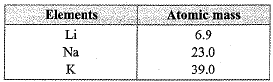Limitation: He could identify only three triads, could not classify all the elements discovered of that time. Elements like N, P, As does not follow Dobereiner’s ‘triads’ law.
Newlands Periodic Table:
Advantage: Every eights element had properties similar to that of first when elements were arranged in increasing order of atomic mass. He correlated properties of elements with atomic mass.
Limitation: It was applicable up to calcium. For example, iron was grouped with oxygen and sulphur whereas it has properties similar to cobalt and nickel.
Mendeleev’s Periodic Table: Advantage: Elements with similar properties could be grouped. He predicted the properties of new elements which helped in their discovery. Disadvantage: No fixed position of hydrogen. Position of isotopes could not be justified . Increasing order of atomic mass could not be maintained.
(b) Henry Moseley
(c) Modern Periodic law: Properties of elements are periodic function of their atomic number.

Q.2. (a) The modern periodic table has been evolved th rough the early attempts of Dobereiner, Newland and Mendeleev. List one advantage and one limitation of all the three attempts.'
(b) Name the scientist who first of all showed that atomic number of an element is a more fundamental property than its atomic mass.
(c) State Modem periodic law.     [CBSE 2018]
Ans.
(a) Dobereiner Attempt : Advantage : The atomic mass of the middle element of the triad was the mean of the other two elements.
Limitation : Dobereiner could identify only three triads.
Newland Attempt :
Advantage : Every eighth element has properties similar to that of the first. Sodium is the eighth element after lithium. These two elements have similar properties.
Limitation : Newlands law of Octaves was found to be applicable only upto calcium. After calcium, every eight element did not possess properties similar to that of the first.
Mendeleev Attempt :
Advantage : The elements were arranged on the basis of their fundamental property, the atomic mass and also on the similarity of chemical properties.
Limitation : No fixed position in the periodic table could be given to hydrogen by Mendeleev classification.
(b) Henry Moseley.
(c) Properties of elements are a periodic function of their atomic number.

Q.3. The atomic number of Cl is 17. On the basis of this information, answer the questions that follow : [CBSE 2017]
(a) W rite the electronic configuration of Cl.
(b) Find the valency.
(c) To which group does it belong ?
(d) Identify the type of ion it will form.
(e) Write down the formula of the compound it forms with other elements.

Ans. (a) Electronic configuration of Cl is 2, 8, 7.
(b) Valency of Cl is 1 as it requires only one electron to complete its octet.
(c) It belongs to group 17 of the modern periodic table.
(d) It w ill form an anion (Cl-) by ga inin g one electron.
(e) It form HCl with H and NaCl with Na.

Q.4. In the following table, the positions of six elements A, B, C, D, E and F are given as they are in the Modern Periodic Table: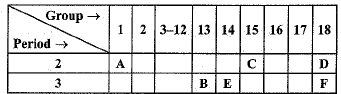On the basis of the above table, answer the following questions:
(i) Name the element which forms only covalent compounds.
(ii) Name the element which is a metal with valency three.
(iii) Name the element which is a non-metal with valency three.
(iv) Out of B and C, whose atomic radius is bigger and why?
(v) Write the common name for the family to which the elements D and F belong.      [Foreign 2015]
Ans. (i) ‘E’ forms only covalent bonds.
(ii) ‘B’ is metal with valency three.
(iii) ‘C’ is non-metal with valency 3.
(iv) ‘B’ is bigger due to less effective nuclear charge.
(v) ‘D’ and T ’ belong to noble gases.

Offer running on EduRev: Apply code STAYHOME200 to get INR 200 off on our premium plan EduRev Infinity!

,

,

,

,

,

,

,

,

,

,

,

,

,

,

,

,

,

,

,

,

,

;